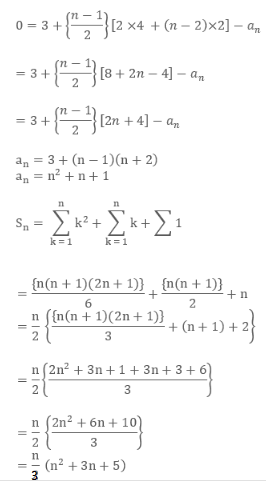# Find the sum of series 3, 7, 13, 21, 31….

Given a number N. The task is to find the sum of below series upto nth term.

3, 7, 13, 21, 31, ….

Examples:

```Input : N = 3
Output : 23

Input : N = 25
Output : 5875
```

## Recommended: Please try your approach on {IDE} first, before moving on to the solution.

Approach:Subtracting the above two equations, we have:Below is the implementation of the above approach:

## C++

 `// C++ Program to find the sum of given series ` ` `  `#include ` `#include ` ` `  `using` `namespace` `std; ` ` `  `// Function to calculate sum ` `int` `findSum(``int` `n) ` `{ ` `    ``// Return sum ` `    ``return` `(n * (``pow``(n, 2) + 3 * n + 5)) / 3; ` `} ` ` `  `// Driver code ` `int` `main() ` `{ ` `    ``int` `n = 25; ` ` `  `    ``cout << findSum(n); ` ` `  `    ``return` `0; ` `} `

## Java

 `// Java program to find sum of ` `// n terms of the given series ` `import` `java.util.*;  ` ` `  `class` `GFG  ` `{  ` `static` `int` `calculateSum(``int` `n)  ` `{  ` `    ``// returning the final sum  ` `    ``return` `(n * ((``int``)Math.pow(n, ``2``) + ``3` `*  ` `                               ``n + ``5``)) / ``3``;  ` `}  ` ` `  `// Driver Code  ` `public` `static` `void` `main(String arr[])  ` `{  ` `    ``// number of terms to  ` `    ``// find the sum ` `    ``int` `n = ``25``;  ` `    ``System.out.println(calculateSum(n));  ` `}  ` `}  ` ` `  `// This code is contributed  ` `// by Surendra_Gangwar `

## Python 3

 `# Python program to find the  ` `# sum of given series ` ` `  `# Function to calculate sum ` `def` `findSum(n): ` `    ``# Return sum ` `    ``return` `(n``*``(``pow``(n, ``2``)``+``3` `*` `n ``+` `5``))``/``3` ` `  `# driver code ` `n ``=` `25` ` `  `print``(``int``(findSum(n))) `

## C#

 `// C# program to find  ` `// sum of n terms of  ` `// the given series ` `using` `System; ` ` `  `class` `GFG  ` `{  ` `static` `int` `calculateSum(``int` `n)  ` `{  ` `    ``// returning the final sum  ` `    ``return` `(n * ((``int``)Math.Pow(n, 2) + 3 *  ` `                               ``n + 5)) / 3;  ` `}  ` ` `  `// Driver Code  ` `public` `static` `void` `Main()  ` `{  ` `    ``// number of terms to  ` `    ``// find the sum ` `    ``int` `n = 25;  ` `    ``Console.WriteLine(calculateSum(n));  ` `}  ` `}  ` ` `  `// This code is contributed  ` `// by inder_verma. `

## PHP

 ` `

Output:

```5875
```

Time Complexity : O(1)

Don’t stop now and take your learning to the next level. Learn all the important concepts of Data Structures and Algorithms with the help of the most trusted course: DSA Self Paced. Become industry ready at a student-friendly price.

My Personal Notes arrow_drop_upCheck out this Author's contributed articles.

If you like GeeksforGeeks and would like to contribute, you can also write an article using contribute.geeksforgeeks.org or mail your article to contribute@geeksforgeeks.org. See your article appearing on the GeeksforGeeks main page and help other Geeks.

Please Improve this article if you find anything incorrect by clicking on the "Improve Article" button below.